# Random forest in python## Introduction to Random forest in python

Random forest in Python offers an accurate method of predicting results using subsets of data, split from global data set, using multi-various conditions, flowing through numerous decision trees using the available data on hand and provides a perfect unsupervised data model platform for both Classification or Regression cases as applicable; It handles high dimensional data without the need any pre-processing or transformation of the initial data and allows parallel processing for quicker results.

Brief on Random Forest in Python:

The unique feature of Random forest is supervised learning. What it means is that data is segregated into multiple units based on conditions and formed as multiple decision trees. These decision trees have minimal randomness (low Entropy), neatly classified and labeled for structured data searches and validations. Little training is needed to make the data models active in various decision trees.

### How Random Forest Works?

The success of Random forest depends on the size of the data set. More the merrier. The big volume of data leads to accurate prediction of search results and validations. The big volume of data will have to be logically split into subsets of data using conditions exhaustively covering all attributes of data.

Decision trees will have to be built using these sub-sets of data and conditions enlisted. These trees should have enough depth to have the nodes with minimal or nil randomness and their Entropy should reach zero.  Nodes should bear labels clearly and it should be an easy task to run through nodes and validate any data.

We need to build as many decision trees as possible with clearly defined conditions, true or false path flow. The end nodes in any decision tree should lead to a unique value.  Each and every decision tree is trained and the results are obtained. Random forest is known for its ability to return accurate results even in case of missing data due to its robust data model and sub-set approach.

Any search or validation should cover all the decision trees and the results are summed up. If any data is missing the true path of that condition is assumed and the search flow continues till all the nodes are consumed. The majority value of the results is assumed in the case of the classification method and the average value is taken as a result in the case of the regression method.

### Examples

To explain the concept of Random Forest a global data set on Vegetables is created using the Python Panda data framework. This data set has high Entropy which means high randomness and unpredictability.

The Code for creating the data set is

```# Python code to generate a new data set import pandas as pd vegdata = {'Name': ['Carrot','Brinjal','Spinach','Spinach','Carrot','Tamato','Tamato','Carrot','Brinjal','Spinach','Tamato','Carrot','Carrot','Brinjal','Brinjal'], 'Colour': ['Red','Green','Green','Green','Red','Red','Red','Red','Green','Green','Red','Red','Red','Green','Green'],'Weather':['Cold','Hot','Cold','Cold','Cold','Hot','Hot','Cold','Hot','Cold','Hot','Cold','Cold','Hot','Hot'],'Weight':[20,20,30,30,20,20,20,20,20,30,20,20,20,20,20] } df = pd.DataFrame(vegdata, columns = ['Name','Colour','Weather','Weight']) print (df) The result is (Global Data set)```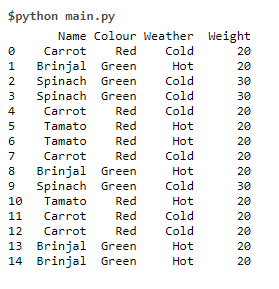The data set need to be split based on conditions. Splitting the data set on Weight = 30 will result in a data set that has Entropy = 0 and it need not be split further.

Data Set 1  Code is print (df.where (df[‘Weight’]==30))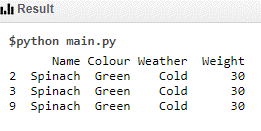The other data set is

Data Set 2  Code is print (df.where (df[‘Weight’]!=30))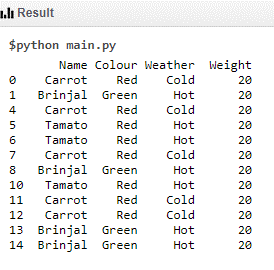It can further be split based on color and the new data set has entropy zero and it need not be split further

Data Set – 3 Code – print (df[(df.Weight == 20) & (df.Colour ==’Green’)])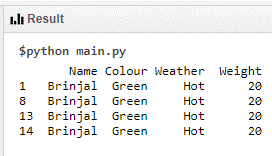The other data set is

Data set – 4 Code – print (df[(df.Weight == 20) & (df.Colour ==’Red’)])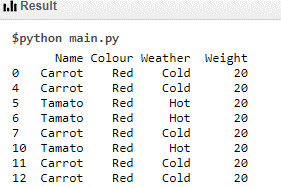It can be further split on Weather and it will result in two data sets which has entropy =0

Data set – 5  Code – print (df[(df.Weight == 20) & (df.Colour ==’Red’) & (df.Weather ==’Cold’)])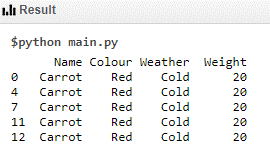Code – print (df[(df.Weight == 20) & (df.Colour ==’Red’) & (df.Weather ==’Hot’)])

Data set – 6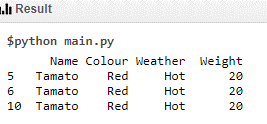Hence a decision tree(No-1) can be formed using the above conditions (Green – true path, Red- false path)

Another decision tree (No-2) can be formed based on the following conditions

Data Set 7  Code – print (df[(df.Colour==’Red’)])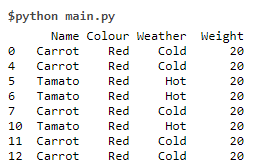Data Set 8 – Code – print (df[(df.Colour==’Green’)])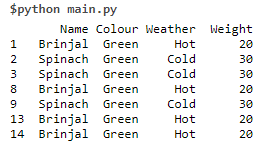Data set 9  Code – print (df[(df.Colour ==’Red’) & (df.Weather ==’Hot’)])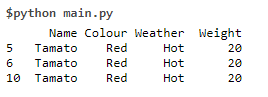Data set 10 Code – print (df[(df.Colour ==’Red’) & (df.Weather ==’Cold’)])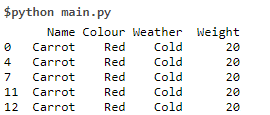Data Set 11  Code – print (df[(df.Colour ==’Green’) & (df.Weather ==’Hot’)])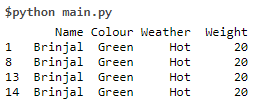Data Set 12 Code – print (df[(df.Colour ==’Green’) & (df.Weather ==’Cold’)])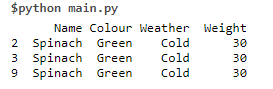Yet Another decision tree (No-3) can be formed based on the following conditions

Global Data Set

Data Set 13  Code – print (df[(df.Weather ==’Hot’)])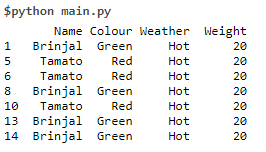Data Set 14  Code – print (df[(df.Weather ==’Cold’)])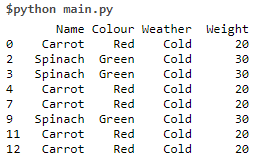Data Set 15  Code – print (df[(df.Weather ==’Hot’) & (df.Colour ==’Red’)])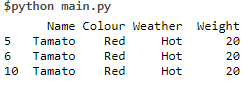Data Set 16  Code – print (df[(df.Weather ==’Hot’) & (df.Colour ==’Green’)])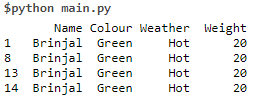Data Set 17  Code – print (df[(df.Weather ==’Cold’) & (df.Colour ==’Red’)])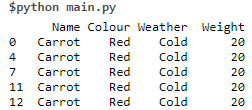Data Set 18  Code – print (df[(df.Weather ==’Cold’) & (df.Colour ==’Green’)])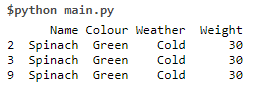We can create a Random forest using the three individual decision trees.

### How to use the Random forest?

If a photo or any other image with a few available data points can be validated with all the 3 decision trees and the possible results are arrived at.

For Example the photo of a vegetable is given for verification with the data of Weight = 18 gram, colour = Red and no other data.

### Steps of search operations

1. In the first decision tree in the root node since its weight is 18 grams, it fails the condition (if the weight = 30) takes the false path (data set -2) and it jumps to data-set-4 due to the presence of colour data. It takes the default true path (grown in cold weather) and the value “Carrot” is arrived at.
2. In the second decision tree at the root node, it takes data set 7 due to its Red colour and takes the default true path (Grown in hot weather) data set 9 and takes vale as “Tomato”.
3. In the Third decision, the tree takes at root node default true path (grown in cold weather) and reaches data set 14. At this node, it takes data set 17 due to the availability of colour data. Data set 17 leads to “Carrot”
4. Majority of value is Carrot and hence the final result is due to the classification method.
5. An averaging method is used in regression method which also predicts the result as “Carrot”

For better results

1. Have more data in the global set. Big data always yield accurate results.
2. Create more decision trees, cover all the conditions
3. Use packages provided by python for creating decision trees and search operations

### Conclusion

The random forest has very high predictability, needs little time to roll out, provides accurate results at the quickest possible time.

### Recommended Articles

This is a guide to Random forest in python. Here we discuss How Random Forest Works along with the examples and codes. You may also have a look at the following articles to learn more –

1. Traceback in Python
2. Knapsack Problem Python
3. sprintf Python
4. Insertion sort in Python

The post Random forest in python appeared first on EDUCBA.

This post first appeared on Best Online Training & Video Courses | EduCBA, please read the originial post: here

# Share the post

Random forest in python

×